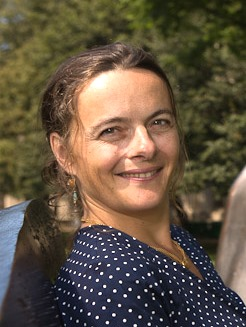distinguished lecture series presents

### MIT

###### Research Area

Probability theory, in particular on large random matrices

###### Visit

Tuesday, May 8, 2018 to Thursday, May 10, 2018

###### Location

Kinsey 1240B/ MS 6627

# Website

## Random matrix theory and Dyson-Schwinger equations

###### abstracts
The uses of Dyson-Schwinger equations: The most well known results from probability theory, such as central limit theorem and the law of large numbers, deal with independnet random variables. Such theorems do not apply to strongly correlated variables such as the eigenvalues of random matrices. To study the fluctuations of such systems the analysis of Dyson-Schwinger equations have been central in the recent years. These equations describe relations between the moments of the observables. they are often derived thanks to the underlying symmetries of the model. In this talk we will introduce Dyson-Schwinger equations in random matrix theory, and their analogue in random tilings given by Nekrasov’s equations. We will describe how to analyze them in order to derive central limit theorems, the universality of local fluctuations or large deviations estimates.
Universality in random matrix theory: In this talk I will focus on universality in Random matrices and joint works with Bekerman and Figalli. To this end, I will introduce the concept of approximate transport maps and show how to construct it thanks to Dyson-Schwinger equations.
Several matrix questions: In this last talk I will discuss Dyson-Schwinger equations for several matrix problems and their applications to obtain central limit theorems and large deviations estimates.
###### Lecture 3
2300 Murphy Hall - Box 951438 - Los Angeles, CA 90095-1438 © 2018